# equilibrium wage? Question 6 1 points Save Answer Consider the following labor market Labor demand: Lap-...

equilibrium wage?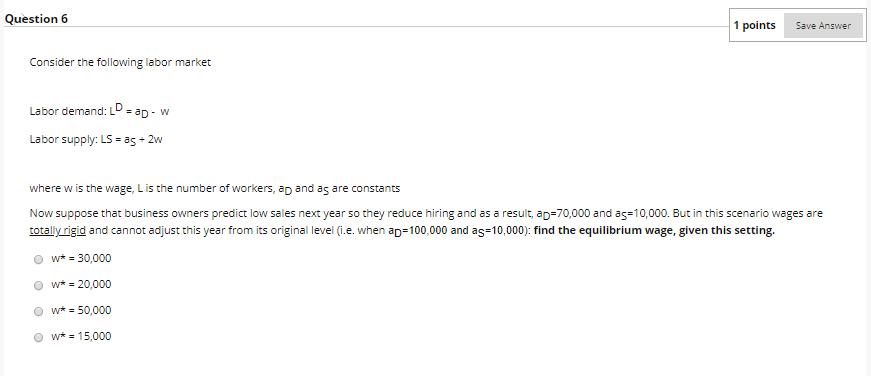Question 6 1 points Save Answer Consider the following labor market Labor demand: Lap- w Labor supply: LS as 2w where w is the wage, Lis the number of workers, ap and as are constants Now suppose that business owners predict low sales next year so they reduce hiring and as a result, ap-70,0000 and as10,000. But in this scenario wages are totally rigid and cannot adjust this year from its orig level (i.e. when ap=100,000 and as10,000): find the equi ibrium wage, given this setting. w*30,000 Ow20,000 Ow50,000 O w-15,000

Initially the market has

Ld = 100000 - w and Ls = 10000 + 2w

This gives

100000 - w = 10000 + 2w

w* = 90000/3 = \$30000

It is given that the equilibrium wage will remain unchanged in both cases. Select the first option, wage = \$30,000.

#### Earn Coin

Coins can be redeemed for fabulous gifts.

Similar Homework Help Questions
• ### Consider the following labor market Labor dernand: LD ap- w Labor supply: LS = as+ 2w...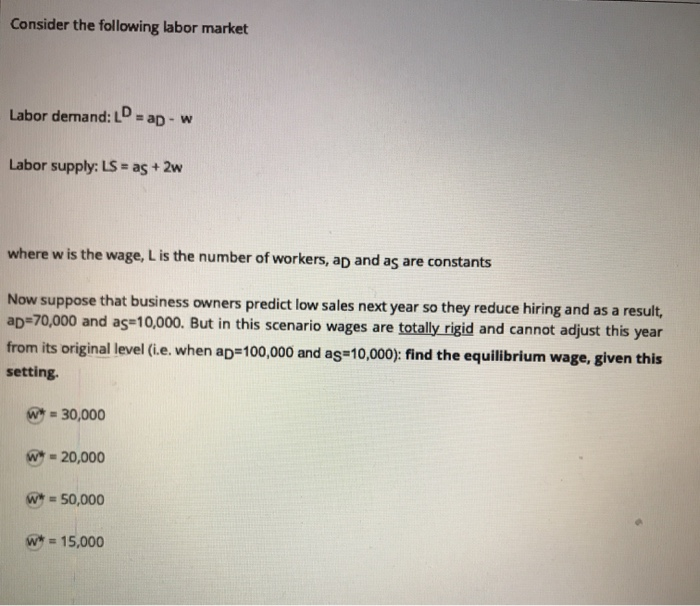Consider the following labor market Labor dernand: LD ap- w Labor supply: LS = as+ 2w where w is the wage, L is the number of workers, ap and as are constants Now suppose that business owners predict low sales next year so they reduce hiring and as a result, ap 70,000 and ag 10,000. But in this scenario wages are totally rigid and cannot adjust this year from its original level (i.e. when ap=100,000 and as10,000): find the equilibrium...

• ### demand and supply Question 7 1 points Save Answer Consider the following labor market Labor demand:...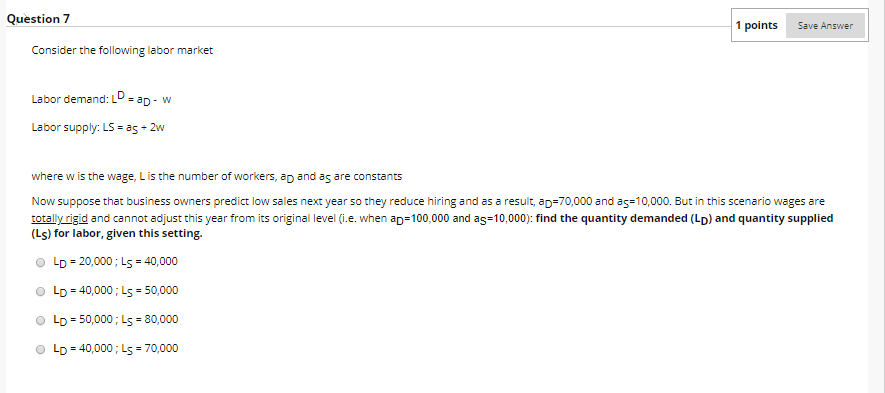demand and supply Question 7 1 points Save Answer Consider the following labor market Labor demand: LD = ad-w Labor supply: LS = as - 2w where w is the wage, L is the number of workers, ap and as are constants Now suppose that business owners predict low sales next year so they reduce hiring and as a result, ap=70,000 and as=10,000. But in this scenario wages are totally rigid and cannot adjust this year from its original level...

• ### Consider the following labor market Labor demand: LD = ap - w Labor supply: LS =...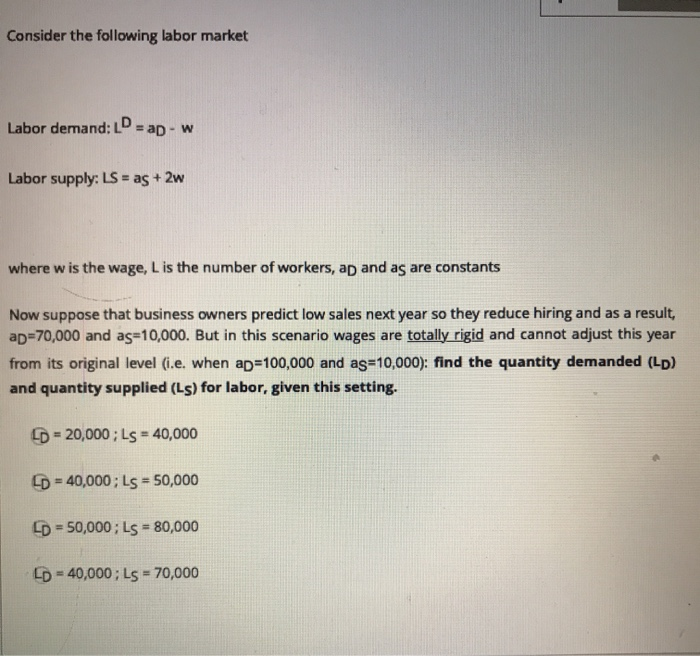Consider the following labor market Labor demand: LD = ap - w Labor supply: LS = as + 2w where w is the wage, L is the number of workers, ap and as are constants Now suppose that business owners predict low sales next year so they reduce hiring and as a result, ap=70,000 and ag=10,000. But in this scenario wages are totally rigid and cannot adjust this year from its original level (i.e. when ap=100,000 and ag=10,000): find the...

• ### What is the equilibrium employment level? Question 2 Consider the following labor market Labor demand: L...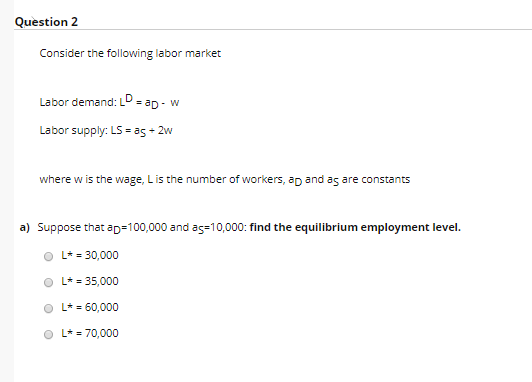What is the equilibrium employment level? Question 2 Consider the following labor market Labor demand: L ap- w Labor supply: LS = as 2w where w is the wage, Lis the number of workers, ap and as are constants a) Suppose that ap 100,000 and as10,000: find the equilibrium employment level L*30,000 L*35,000 L60,000 L70,000

• ### where w is the wage, L is the number of workers, aD and aS are constants...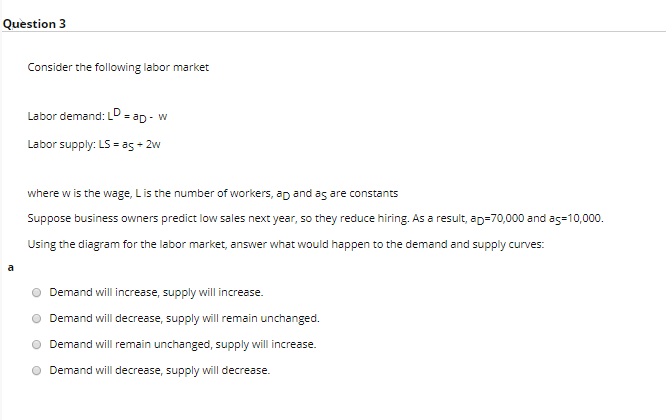where w is the wage, L is the number of workers, aD and aS are constants Suppose business owners predict low sales next year, so they reduce hiring. As a result, aD=70,000 and aS=10,000. Using the diagram for the labor market, answer what would happen to the demand and supply curves: Question 3 Consider the following labor market Labor demand: LD - ad-w Labor supply: LS = 35 + 2w where w is the wage, L is the number of...

• ### Problem #4: Own-price elasticity Suppose the market labor demand curve is given by LD 20- (1/2)W...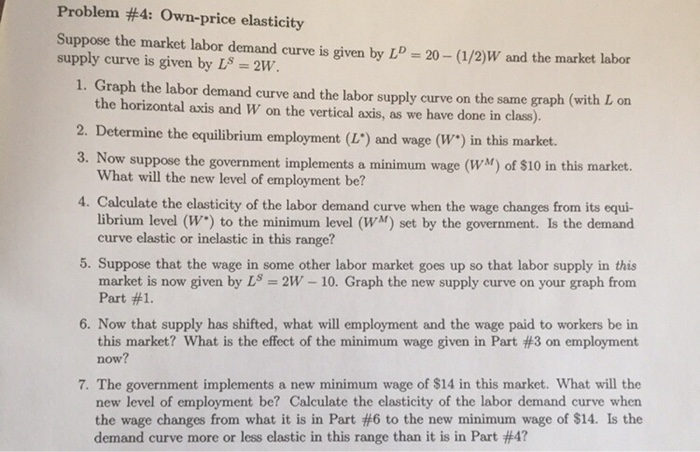Problem #4: Own-price elasticity Suppose the market labor demand curve is given by LD 20- (1/2)W and the market labor supply curve is given by LS-2W 1. Graph the labor demand curve and the labor supply curve on the same graph (with L on the horizontal axis and W on the vertical axis, as we have done in class). 2. Determine the equilibrium employment (L") and wage (W") in this market. Now suppose the government implements a minimum wage (WM)...

Free Homework App